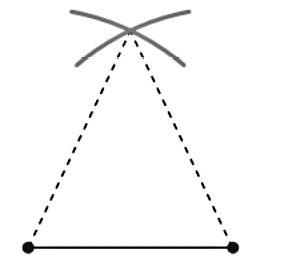Chapter 1.2, Problem 29E### Elementary Geometry for College St...

6th Edition
Daniel C. Alexander + 1 other
ISBN: 9781285195698

#### Solutions

Chapter
Section### Elementary Geometry for College St...

6th Edition
Daniel C. Alexander + 1 other
ISBN: 9781285195698
Textbook Problem
1 views

# On a piece of paper, use your compass to construct a triangle that has two sides of the same length. Cut the triangle out of the paper and fold the triangle in half so that the congruent sides coincide (one lies over the other). What seems to be true of two angles of that triangle?To determine

To understand:

That what seems to be true of the two angles of the triangle which is formed on a piece of paper with two sides of same length.

Explanation

Calculation:

Given,

When the triangle is folded to a triangle in half so that the congruent sides coincide,

### Still sussing out bartleby?

Check out a sample textbook solution.

See a sample solution

#### The Solution to Your Study Problems

Bartleby provides explanations to thousands of textbook problems written by our experts, many with advanced degrees!

Get Started

#### Rationalize the denominator: x12x.

Applied Calculus for the Managerial, Life, and Social Sciences: A Brief Approach

#### Differentiate the function. f(x) = x ln x x

Single Variable Calculus: Early Transcendentals, Volume I

#### Evaluate dx using the Fundamental Theorem of Calculus. a) 7 b) 9 c) d) 18

Study Guide for Stewart's Single Variable Calculus: Early Transcendentals, 8th

#### Solve 34E+(18E)=214E+12 .

Mathematics For Machine Technology